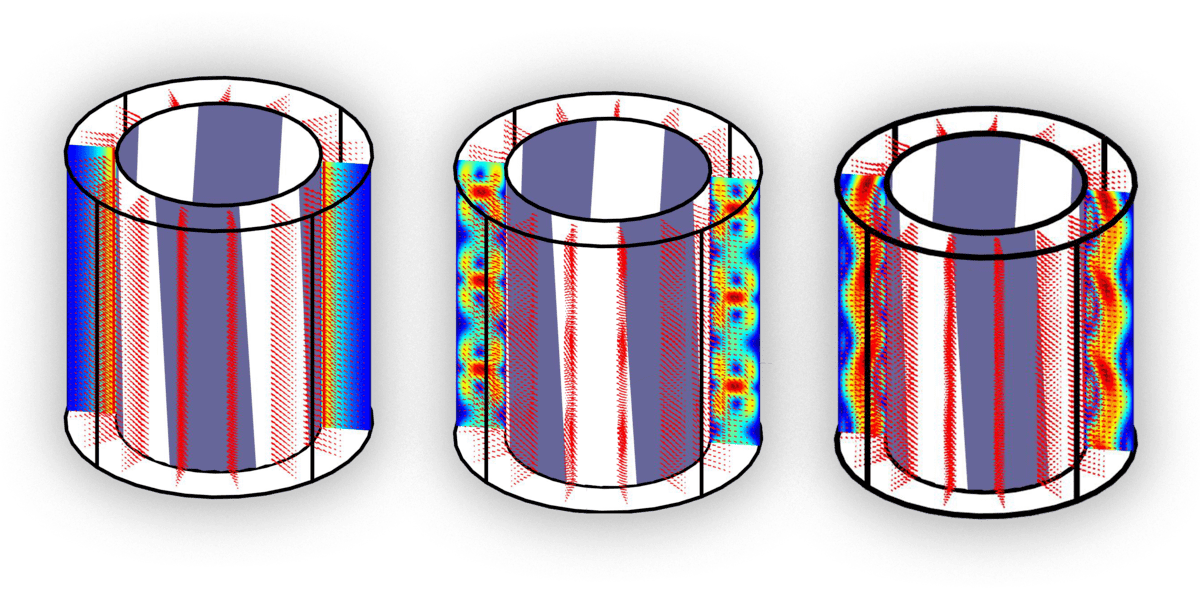# Any packages for fluid simulation?!

6 views (last 30 days)
Rozi Sh on 14 Jun 2011
Edited: Precise Simulation on 5 Nov 2021
Hi! I want to simulate a fluid in a rotating cylindrical container (Infinite long cylinder). In the fluid there exist small objects too. I have no idea about the package that can help me.

Walter Roberson on 14 Jun 2011
You want to simulate an infinitely large chaotic process in a finite amount of memory??
The small objects: are they anchored? If not, how buoyant are they compared to the estimated lifting ability of the fluid? If they aren't anchored then there may be gravitational or electrostatic attractions between the objects, or the wave motion might happen to force them in to proximity: once in proximity, the viscosity of the fluid could act to "drag" them with respect to each other, resulting in one "climbing" on the other. Such effects would, it seem to me, require quite a fine-grained simulation, but the finite amount of memory you have for the infinite simulation suggests you will not be able to provide that granularity. Or are you planning on fine-scale simulation within a certain distance of the objects and mere statistical non-chaotic calculations beyond that range?
What model of the geometry of the Universe are you planning to use? If you model it according to the current models of the curvature of the Universe, then your infinite cylinder might have to be a closed torus -- but a closed torus has different wave propagation modes than a cylinder does. If you instead model the Universe as infinite and linear and as containing an infinite amount of matter (an assumption needed in order to be able to fill the infinite cylinder with fluid), then I suspect that it could be shown that the probability is 1 that some portion of the cylinder will pass close enough to the gravitational influence of a massive object (star, black hole, significant planet) that you will need to include gravitational influence in that area of the cylinder.
Thomas Clark on 8 Aug 2012
With no sarcasm at all, I'll simply state that an infinitely long cylinder can be trivially modelled with periodic boundary conditions at either end of a finite domain.
Moreover, since there is no information about Reynolds Number we cannot state that the problem is chaotic - it may even be sufficiently represented by a 2D setup.
In a more useful response to the OP, try OpenFOAM, which is a CFD solver which should be able to handle the type of problem you're dealing with. MATLAB doesn't have flow solvers.
I'll warn you, however - guessing from your level of detail in the question that you really don't know what you're trying to do yet - and OpenFOAM (CFD in general) is NOT for the faint hearted. There is a huge learning curve ahead so figure out exactly what you want first.
Good luck.

Precise Simulation on 6 Jul 2019
Edited: Precise Simulation on 5 Nov 2021
For future reference, the linked tutorial below
shows how to set up and perform MATLAB CFD simulation of axisymmetric (swirling) Taylor-Couette flow with both a simple toolbox GUI and the MATLAB CLI interface
: :